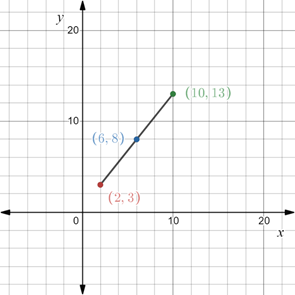# To graph : The line segment AB where coordinates of the point A are ( 2 , 3 ) . Also the point M ( 6 , 8 ) is the mid-point of the line segment and compute the coordinate of the point B .### Precalculus: Mathematics for Calcu...

6th Edition
Stewart + 5 others
Publisher: Cengage Learning
ISBN: 9780840068071### Precalculus: Mathematics for Calcu...

6th Edition
Stewart + 5 others
Publisher: Cengage Learning
ISBN: 9780840068071

#### Solutions

Chapter 1.8, Problem 118E
To determine

## To graph: The line segment AB where coordinates of the point A are (2,3) . Also the point M(6,8) is the mid-point of the line segment and compute the coordinate of the point B .

Expert Solution

### Explanation of Solution

Given information:

AB is a line point and M is mid-point of the line segment.

Graph:

Plot the points A(2,3) and M(6,8) on the coordinate plane.

Let the coordinates of the point B be (x,y) .

Recall that the midpoint of the segment that joins two points A=(x1,y1) and B=(x2,y2) in the Cartesian plane is denoted by (x1+x22,y1+y22) .

Now, M(6,8) is the mid-point of the line segment joined by the points A(2,3) and B(x,y) .

Therefore,

(2+x2,3+y2)=(6,8)

Equate the x -coordinate of each bracket,

2+x2=62+x=12x=10

Equate the y -coordinate of each bracket,

3+y2=83+y=16y=13

Therefore, coordinates of the point B are (10,13) .

Now, plot the points A(2,3) , B(10,13) and M(6,8) on the coordinate plane.Interpretation:

As the point M(6,8) is the midpoint of the line segment joined by the point A(2,3) and B(10,13) , it is observed that the distance point the points A(2,3) and M(6,8) is same as the distance between the points B(10,13) and M(6,8) .

### Have a homework question?

Subscribe to bartleby learn! Ask subject matter experts 30 homework questions each month. Plus, you’ll have access to millions of step-by-step textbook answers!